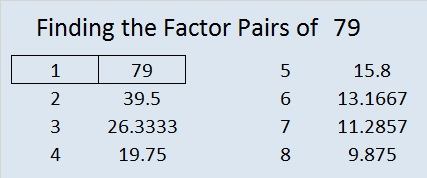# 79 and Level 3This week’s puzzles and last week’s solutions: 10 Factors 2014-03-31

• 79 is a prime number.
• Prime factorization: 79 is prime.
• The exponent of prime number 79 is 1. Adding 1 to that exponent we get (1 + 1) = 2. Therefore 79 has exactly 2 factors.
• Factors of 79: 1, 79
• Factor pairs: 79 = 1 x 79
• 79 has no square factors that allow its square root to be simplified. √79 ≈ 8.8881944How do we know that 79 is a prime number? If 79 were not a prime number, then it would be divisible by at least one prime number less than or equal to √79 ≈ 8.8. Since 79 cannot be divided evenly by 2, 3, 5, or 7, we know that 79 is a prime number.

79 is never a clue in the FIND THE FACTORS puzzles.

A Logical Approach to find the factors: Find the column or row with two clues and find their common factor. Write the corresponding factors in the factor column and factor row.  Because this is a level three puzzle, you have now written a factor at the top of the factor column. Continue to work from the top of the factor column to the bottom, finding factors and filling in the table one row at a time as you go: